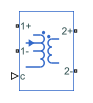# Tap-Changing Transformer

Single-phase tap-changing transformer

•Libraries:
Simscape / Electrical / Passive / Transformers

## Description

The Tap-Changing Transformer block represents a single-phase tap-changing transformer. You can vary the turns-ratio of the transformer during the simulation using the control input.

Use this component to regulate or change the output voltage of a linear transformer during a simulation. To model the effects of saturation, consider using the Nonlinear Transformer block.

### Operating Principle

Use the control input c to change the tap position of the transformer.

• Increase c above the Control threshold, t, to increase the tap position p by one.

• Decrease c below the negative of the Control threshold to decrease the tap position p by one.

This figure shows the tap-response to a control input.In the diagram:

• N0 is the nominal number of turns for the tap-changer winding.

• δ is the percent change in turns per tap position step. Specify this value using the Change per tap (%) parameter.

• pmin and pmax are the minimum and maximum allowable tap indices. Specify these values with the Minimum tap index (nominal=0) and Maximum tap index (nominal=0) parameters.

To select the tap-changer winding, use the Tap-changer location parameter. The overall turns ratio, n, depends on this location:

• `Primary`

`$n=\frac{{N}_{p}\left({N}_{0},\delta ,p\right)}{{N}_{s}}={n}_{0}\left(1+\frac{\delta p}{100}\right)$`

• `Secondary`

`$n=\frac{{N}_{p}}{{N}_{s}\left({N}_{0},\delta ,p\right)}={n}_{0}{\left(1+\frac{\delta p}{100}\right)}^{-1}$`

where n0 is the nominal turns ratio for the transformer.

### Equivalent Circuit

The equivalent circuit is shown in the diagram.Here:

• Rp and Rs are the primary and secondary series resistances, respectively. Changes in the tap position affect these values.

• Llp and Lls are the primary and secondary leakage inductances, respectively. Changes in the tap position affect these values.

• Rm and M are the magnetization resistance and inductance, respectively. Changes in the tap position affect these values.

• n is the turns ratio of the transformer.

• Gp and Gs are the primary and secondary leakage conductances, respectively. Changes in the tap position do not affect these values.

## Ports

### Conserving

expand all

Electrical conserving port associated with the positive terminal of line 1.

Electrical conserving port associated with the negative terminal of line 1.

Electrical conserving port associated with the positive terminal of line 2.

Electrical conserving port associated with the negative terminal of line 2.

Input physical signal that specifies the input control value.

## Parameters

expand all

### Nominal

Turns ratio of the transformer in the nominal tap position. The turns ratio is defined as the primary number of turns divided by the secondary number of turns.

Leakage inductance of the primary winding.

Leakage inductance of the secondary winding.

Mutual resistance of the transformer.

Magnetization inductance of the transformer.

Series resistance of the primary winding.

Series resistance of the secondary winding.

Leakage conductance of the primary winding. Set this value to be nonzero for achieving numerical convergence in some circuit topologies.

Leakage conductance of the secondary winding. Set this value to be nonzero for achieving numerical convergence in some circuit topologies.

### Tap

Specify whether the tap-changer is on the primary or secondary winding.

Minimum allowable position of the tap-changer.

Maximum allowable position of the tap-changer.

Percent change in the number of turns per tap position step of the tap-changer winding. Set this value such that the absolute percent change at the minimum and maximum tap indices is less than `100` `%`.

Control value at which the tap changes position. To lower the tap position, apply a control signal c smaller than the negative of this value. To increase the tap position, apply a control signal c greater than this value.

## Version History

Introduced in R2018a